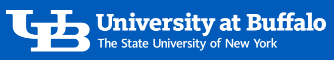### Let's get started with a program that uses variables and methods!

• Open Eclipse
• Right-Click on your `buffalo.edu.fa23.` package
• Choose: `File -> New -> class`
• In the `Name:` textbox type: `Triangle`
• Click: `Finish`
• Copy the `Triangle.java` Template (Java) code (below)
• Paste that code into your new Triangle class in the editor
• Change both occurrences of put-your-lastname-here
• Replace the blank on the
`private int`
line with:
`S1, S2, S3`
• After the
`public Triangle ( int s1, int s2, int s3 ) {`
```S1=s1;   S2=s2;   S3=s3;```
`public int calculatePerimeter( ) {`
`return (S1+S2+S3)`
`Triangle t = new`
`Triangle(10,5,2)`